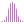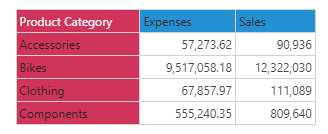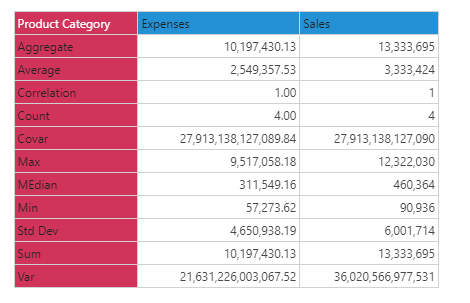#Stdev (PQL)

Returns the sample standard deviation across elements in the specified list.

#### Syntax

Stdev( <List> , OPTIONAL )

* Click on the function's arguments above for more details on the input values.

• There are 2 variants of the function: one for sample and one for the population ("P").
• List parameter should be a list or set of elements from an existing hierarchy in the model.
• The data point or tuple is optional, and describes the values used to generate the standard deviation . If not supplied, the default measure or the query context will determine this value instead.
• For details on how to employ and use this function see the semantic calculation overview.
##### Different Function types
• This function is like the MDX sample standard deviation function; it calculates the standard deviation across a list of elements in a hierarchy or attribute.
• To use a simple standard deviation mathematical operation on a list of numbers, use the Common library function.

#### Examples

This example returns the sample standard deviation (sample math) of expenses across the product category in the product table/ dimension, using the sample demo model:

StDev( {AllMembers([products].[Product Category])}, ([measures].[data Expenses]) )

Below, using the sample data of the 4 product categories we can use the above formula to calculate the sample standard deviation. Looking at the list below, the standard deviation value for expenses is 4,650,938: